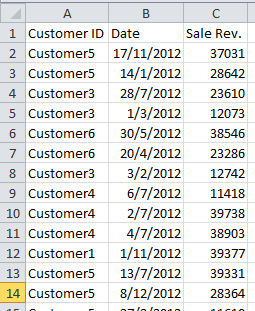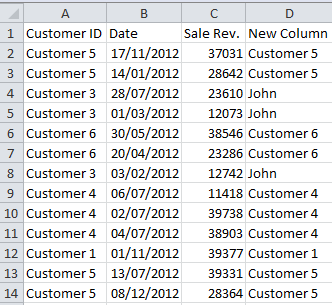# Excel If Formula

Excel If function lets you make calculations or selections based on conditions. Excel if formula is likely one of the most used formulas in Excel. From calculations to formatting, you will use is in a lot of occasions.

Let’s say you have a list of sales shows sales transactions from several customers and you want to change customer name for customer3. To do this;

• you need to create a helper column
• write an excel if formula that checks customer names
• and changes it if customer name is “customer3”.

Here is how it is done:

Our example data is like picture below:For this task we are going to make a helper column in column D (write Helper in D1). And we are going to write an if formula in D2 cell. If formula will be like this:

=if(A2=”Customer3″;”John”;A2)

This Excel if formula says “if value of cell A2 equals to Customer3, make it John, else make it cell A2’s value”. As you can see syntax of excel if function is:

=if(condition check ; do this if condition returns true ; do this if condition returns false)

When you apply this if formula for in D column for every row of the list, you will have a new alternative Customer ID column that shows same values as Column A but shows John for Customer3.